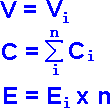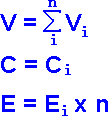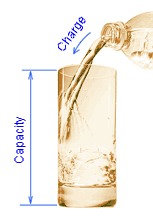# Battery Capacity - what is it?

## 1. Battery Capacity - the most valuable battery charachteristic

Battery capacity shows how long the battery after full charge can feed the load connected to the battery. Usually the unit of battery capacity is ampere-hous (Ah) or milliampere-hours (mAh) for small batteries.

The unit of measurement itself shows that battery capacity is the product of constant current flowing through the load connected to a battery (in amps or in milliamps) and the discharge time in (hours).

C [A*h] = I [A] x T [h]

## 2. Battery capacity and battery energy

Sometimes people think that battery capacity is the characteristic of total energy that can be stored inside the battery. It is not so because the energy depends on battery voltage - the more is battery voltage the more is energy stored in the battery at full charge. As we know electrical energy is the product of voltage, current and the time of current flow.

E [J] = I [A] x U [V] x T [s]

That is why the battery energy (in watt-hours) is the product of battery capacity and battery nominal voltage.

E [Wh] = C [Ah] x Unom [V]

## 3. Battery capacity and battery energy for batteries connected in parallel or in seriesBattery parallel
connection lawsBattery serial
connection laws

If several batteries of the same capacity are connected in series the capacity of this large battery is the same as the capacity of single battery. The energy of the large battery is the product of energy of a single battery and number of batteries connected in series.

If several batteries of the same voltage are connected in parallel the capacity of this large battery is the sum of the capacity of all batteries. The energy of the large battery is the product of energy of a single battery and number of batteries connected in series.

## 4. What other parameters do charactirize battery capacity

### Energy Capacity

So called energy capacity shows the constant power with which the battery can discharge during some short period - usually 15 minutes (check this time carefully - some manufacturers are trying to confuse customers with 10 min period). Energy capacity is measured in watts per cell or (sometimes) in watts per battery.

One can roughly estimate the nominal (C10 or C20) capacity of a battery with given energy capacity by:

C [Ah] = W [W/cell] / 4

### Reserve Capacity

One of the characteristics of car lead-acid battery is reserve capacity. Reserve capacity shows how long a car battery can feed car loads if car generator is not working. Reserve capacity is measured in minutes of battery discharge with 25 A current.

One can roughly estimate the nominal (C10 or C20) capacity of a battery with given reserve capacity by:

C [Ah] = T [min] / 2

## 5. Battery capacity and battery charge (battery state of charge)Battery capacity
and battery charge
(analogy)

Sometimes people are confusing battery capacity and battery charge. Battery capacity shows the potential of a battery - it characterizes how long the battery would be able to supply the load if it is fully charged. Charge characterizes to which percentage this potential is used just now.

We can compare it with a glass of water. The glass volume (capacity) does not depend on the amount of liquid that the glass contains just now. So is a battery - charged or discharged, battery has the same capacity.

## 6. What does battery capacity depend on?

### Discharge Current

Usually a manufacturer of lead-acid battery assigns as nominal capacity the capacity during prolonged (10, 20 or 100 hours) discharges. This capacity is denoted by C10, C20 or C100, respectively. The current that flows through the load during 20-hour discharge is denoted by I20. If we know the battery capacity we can calculate this current:

I20 = C20 [Ah] / 20 [h]

Does it mean that I0.25 (current of 1/4 hour discharge) equils C20 x 4 ? No, it is not correct. Lead-acid battery capacity for 15-minute (1/4 hour) discharge usually is slightly less then half of C20. That is why I0.25 is not more then C20x2. As we see discharge current and discharge time ore not directly proportional.

The dependence of the discharge time of the discharge current is close to a power. The formula describing this relationship is known as the Peukert law after German scientist of 19-th sentury. Peukert found that for any battery:

Ip x T = const

Here p is a Peukert number - an exponent that is constant for a given battery or a given battery type.

A Peukert number for lead-acid batteries is usually between 1.15 and 1.35. One can count Peukert number basing on nominal battery capacity. If we know Peukert number we can calculate battery capacity for any given discharge current I:

C = Cnom x (I / Inom)p

Here Cnom is nominal capacity (C10, C20 or C100); Inom - current for which the nominal capacity is assigned (I10, I20 or I100).

### Final Discharge Voltage

Batery voltage decreases while battery discharges. Battery discharge should be stopped when battery voltage falls below some pre-designated voltage - so called final discharge voltage (or cut off voltage). The less is the final dischatge voltage the more is battery capacity. Battery manufacturer assignes the absolute minimum final discharge voltage (dependant of discharge current). If battery is discharged below this point the battery can be damaged due to overdischarge.

### Temperature

Nominal batery capacity is usually rated at some standard temperature (most often 20°C=68°F of 25°C=77°F). If battery temperature rizes from 20°C to 40°C (104°F) lead-acid battery capacity increases by about 5%. With temperature decreasing from 20°C to 0°C (32°F) lead-acid battery capacity is reduced by about 15%. As the temperature decreases by 20°C (68°F), the lead-acid battery capacity falls by another 25%.

### Battery depreciation (aging)

When lead-acid battery is delivered it's capacity may be slightly more or slightly less than the rated (nominal) capacity. After several cycles of discharge-charge or a few weeks at a "floating" charge the battery capacity increases. With further use or storage battery capacity falls - the battery wears out, gets older and eventually needs to be replaced with a new battery. To replace the battery in time one needs to watch battery capacity degradation with the help of modern battery capacity tester.